Latest Banking jobs   »   Quantitative Aptitude Quiz For IBPS PO...

# Quantitative Aptitude Quiz For IBPS PO Mains 2022-20th January

Directions (1-3): Working efficiency of A is 20% more than that of B. B can complete a work ‘X’ in 36 days.
B and C together started to complete the work ‘X’ and after 10 days they both left the work and then remaining work is done by A alone in 15 days.
A and C together started to complete another work ‘Y’ and after working for 12 days they both left the work. Remaining work is done by B alone in 16 days. D first completed work ‘X’ and then completed work ‘Y’ in total 38 days.
It is given that efficiency of all, in completing work ‘X’ and work ‘Y’ is same.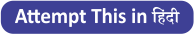Q1. A, B and C working together completed ⅓rd of work ‘X’, and then A and C are replaced by D. Now remaining of work ‘X’ is completed by B and D together. For how many days B worked?
(a) 12 days
(b) 10 days
(c) 15 days
(d) 4 days
(e) 8 days

Q2. A, C and D working simultaneously completed work ‘X’ in ‘n’ days and A, B, C and D working simultaneously completed work ‘Y’ in ‘m’ days. Find the value of (m+n).
(a) 15 days
(b) 10 days
(c) 12 days
(d) 8 days
(e) 17 days

Q3. A person E starts the work ‘X’ and leave after 12 days, then B and C complete the remaining work in 8 days. What is the ratio of number of days taken by A and E together to complete the work ‘X’ to the number of days taken by D, B and C together to complete the both work ‘X’ and ‘Y’ .
(a) 3 : 5
(b) 5 : 3
(c) 8 : 7
(d) 1 : 2
(e) 2 : 3

Directions (4-5): Abhishek bought some chairs and tables from a shopkeeper. The marked price of a chair and a table were in the ratio 5 : 8. The shopkeeper gave discounts of 20% and 25% on the chair & the table respectively. The ratio of number of chairs and tables bought by Abhishek is 6 : 5.

Q4. If Abhishek sells each chair and table bought by him at discounts of 25% and 20% respectively after marking up the prices of both by 50% and gives one table free for every four chairs bought by a customer and only ⅔rd of the total chairs are sold in bunch of four chairs, then what is the net profit /loss % made by Abhishek after selling all of the items which he bought from the shopkeeper?
(a) 6⅔%
(b) 3⅓%
(c) 2 ½ %
(d) 4 ¼ %
(e) 5 %

Q5. If the marked price of a table set by the shopkeeper was Rs.300 more than that of a chair and the total expenditure made by Abhishek in purchasing the chairs and table from the shopkeeper was Rs.108000, then how many chairs were purchased by Abhishek?
(a) 150
(b) 60
(c) 120
(d) 90
(e) 80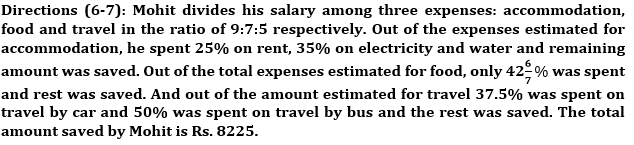Q6. What is the difference of the amount spent on food (excluding saving) and the amount spent on travel by car by Mohit?
(a)Rs 3500
(b)Rs 2520
(c)Rs 1125
(d)Rs 2550
(e)Rs 2250

Q7. Mohit’s total salary is?
(a)Rs 22000
(b)Rs 24000
(c)Rs 26000
(d)Rs 21000
(e)Rs 23000

Directions (8-10): There are three sections A, B and C in a class. Every section has some boy and some girl students in it. Probability of a girl being selected when one student is selected randomly from section A is 2/5, that from section B is 4/9 and that from section C is 5/9.

Q8. If the ratio of total number of students in sections A, B and C is 10 : 12 : 9, then what is the probability of a girl being selected when one student is selected randomly from the students from all the three sections together?
(a) 14/31
(b) 11/23
(c) 13/31
(d) 43/93
(e) Cannot be determined

Q9. If the number of girls in sections A is same as the number of boys in section C, then what is the ratio of number of boys in section A to the number of boys in section C?
(a) 2 : 3
(b) 3 : 4
(c) 3 : 2
(d) 4 : 3
(e) 3 : 5

Q10. If 20 girls leaves section B, then the probability of a boy being selected when one student is selected randomly from this section will be 5/8. What is the number of boys in section B?
(a) 120
(b) 100
(c) 75
(d) 60
(e) Cannot be determined

Solutions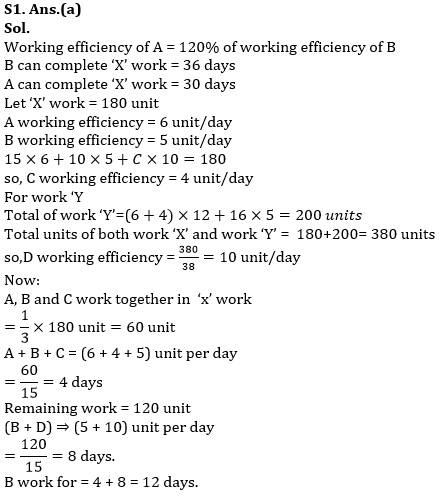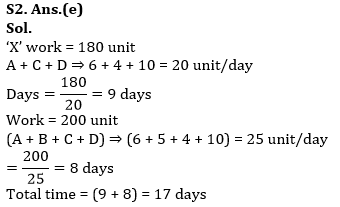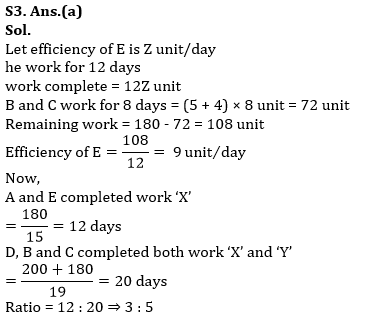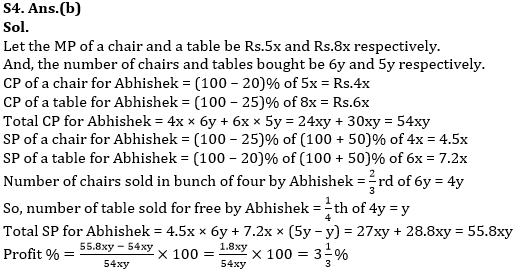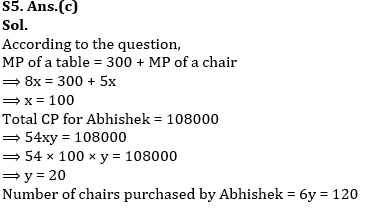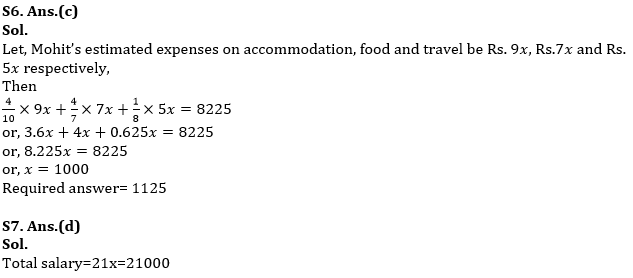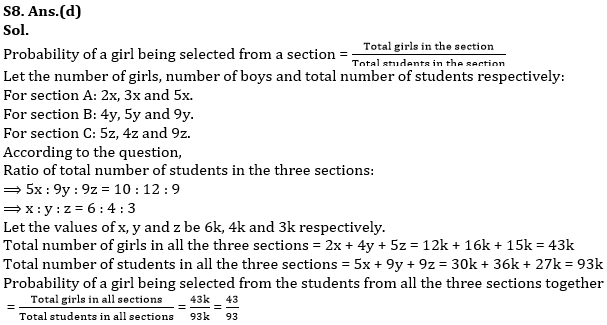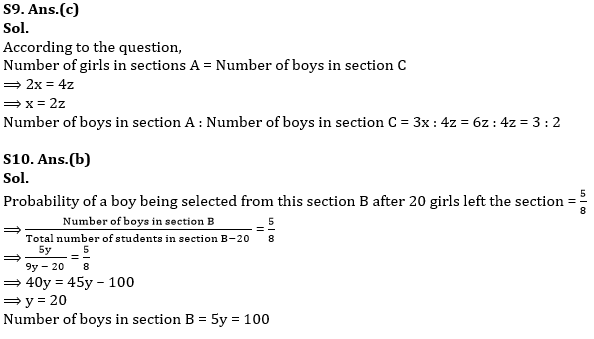#### Congratulations!